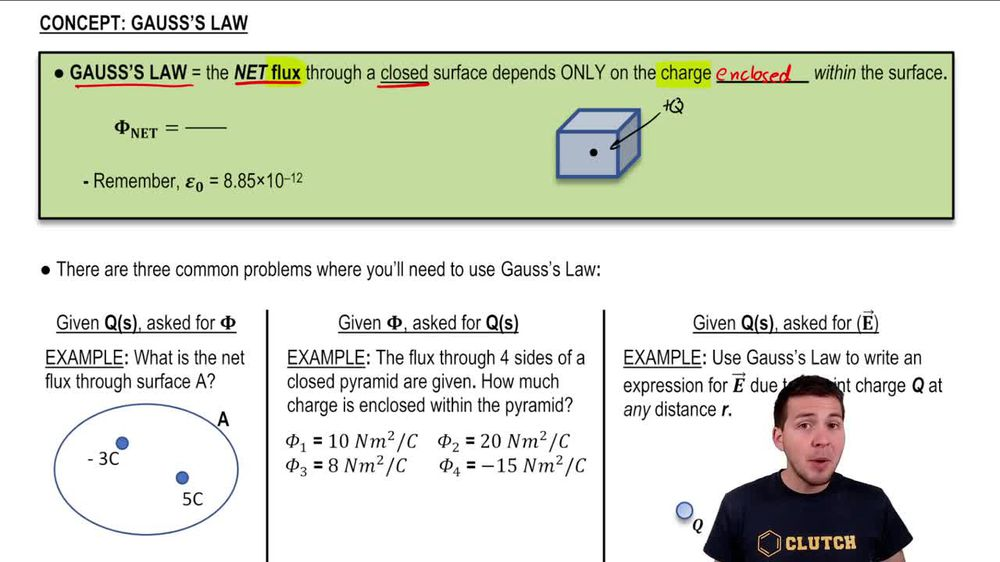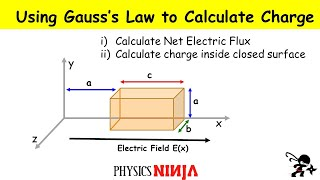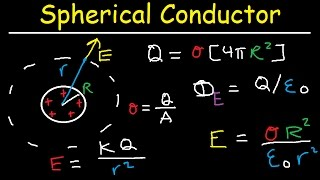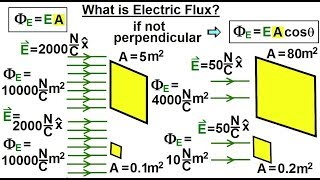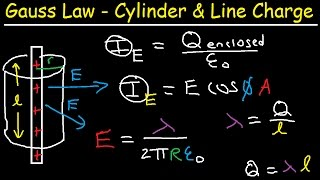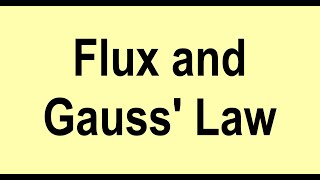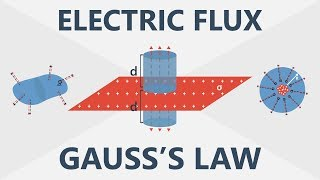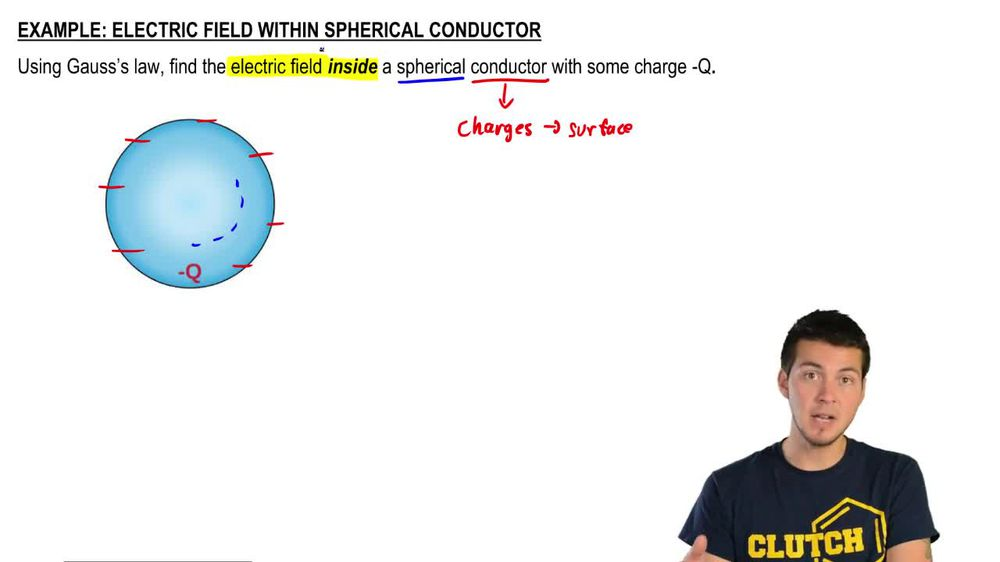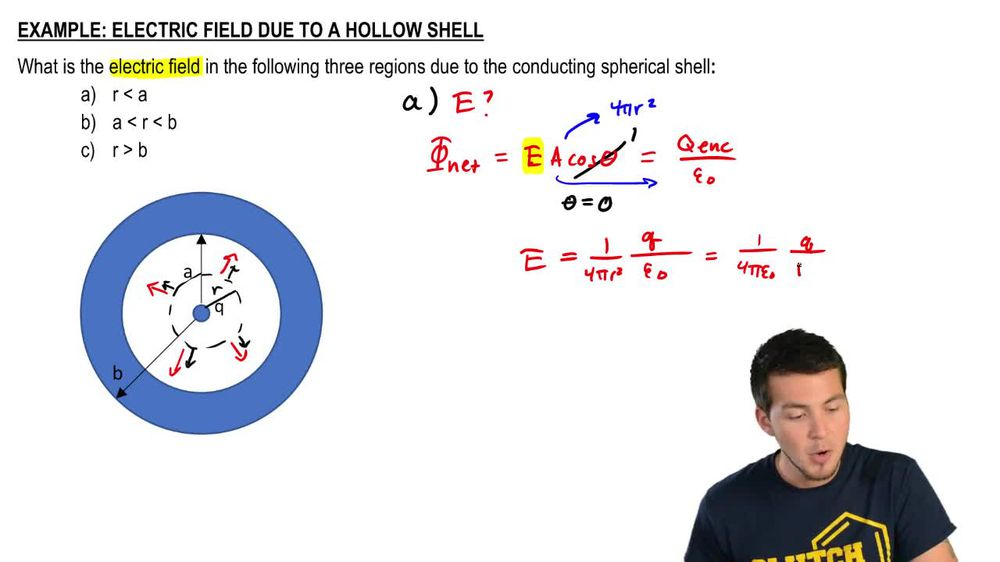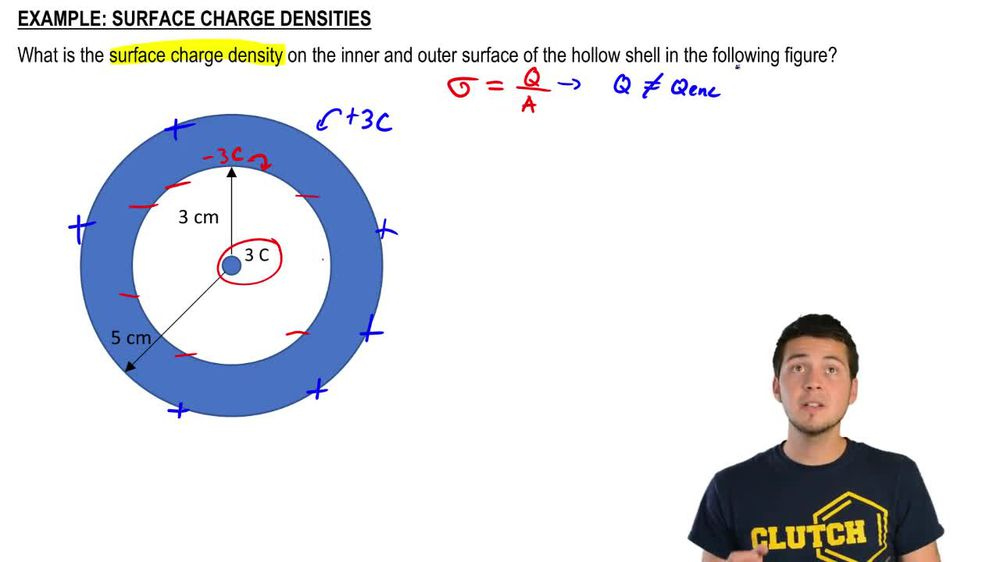Start typing, then use the up and down arrows to select an option from the list.
1. 24. Electric Force & Field; Gauss' Law2. Gauss' Law# Surface Charge Density

by Patrick Ford
327 views
3
1
3
Alright, guys, welcome back. We're gonna work out this example together. We're supposed to figure out what the surface charge density is on the inner and outer surfaces. Now, before you watch this, just make sure that you've seen the last video on how to figure out what the electric fields are at different points throughout this conducting surface. Because you need to know what happens with the charges that are set up on the inside walls, things like that. Just make sure you watch the last video and you're good to go. So we do know from last video that due to gas is law. There's an electric field inside of here, the electric field zero within the conductor, and that exists again on the outside. And this is because whatever charge exists over here gets set up and basically distributed around the inner walls of the inside of this conductor. Here. We know that if this is three cool homes thing, this has to be a negative three cool arms that gets set up on the inner walls. And then on top of that, there has to be a positive three cool homes that gets set upon the outer walls because of charge conservation, right? So I'm just gonna use plus signs everywhere. So basically we're supposed to dio is what was supposed to figure out what the surface charge density is on these inner surfaces. Now that surface charge density is given by the letter Sigma and that Sigma has an equation. It's the total amount of charge divided by the total amount of area. So the I want to be very careful here because this Q is not the enclosed charge of like what we using Gaussian surfaces. So Q is not the Q enclosed. Where we're basically doing is we're just figuring out Okay, If all of these charges here get distributed on the surface of this tiny little thin shell right here on the inside walls of this conductor, then basically, what is the total amount of charge divided by the total amount of area here? So just don't make the mistake and saying that this is the total amount of enclosed charge that's different. So Thesiger MMA, we're gonna have to figure out what the charges are and divided by the areas. All right, so that means that sigma on the inside wall is gonna be the total amount of of charge that's on the inside wall. In other words, the negative three columns divided by the area of the inside wall. Now the area of this inside wall here is just equal to four pi. Times are inside squared and we actually have with the surfaces are we know that each of these we know this is three centimeters and this is five centimeters in the outer wall. In other words, a inside is just four pi, and now we have to do three centimeters. So we have to convert that we have to square that. So that means the area of the inside wall is going to be. I've got 1.13 times 10 to the minus two. That's in square meters. So basically, I can go ahead and just plug that in here and figure out what the surface charge density is. So Sigma on the inside is gonna be Q, which is negative three cool OEMs divided by 1.13 times 10 to the minus two and my sigma inside equals. Let's see, I've got negative 265 and that's gonna be cool OEMs per meter squared, right? So that's basically how you figure out what the surface charge density of the inside wall is now. To do the same thing on the outside wall is just gonna be the exact same process. So to figure out what Sigma out is that's just gonna be cube divided by a the outside wall. So this a of the outside is just gonna be four pi. And then now we just use instead of using three centimeters, we're just gonna use five centimeters. I'm gonna plug that in. So I've got 0.5 and then we're gonna square that. So that means that this outside area is just equal to, uh, let's see, I've got 3.14 times, 10 to the minus two, and that's just gonna go straight in this equation right here. Okay, So that means that the outside surface charge density is just the let's see, we've got three cool owns distribute on the outside divided by the area, which is 3.14 times 10 to the minus two, and that is equal to 95 cool OEMs per meter squared. Notice how this surface charge density ends up being positive, and this one ends up being negative because that actually has to do with the amount of charge that's divided by this area here. So those charges actually could be negative or positive. All right, let me know if you guys have any questions with this, I'll see you the next one.